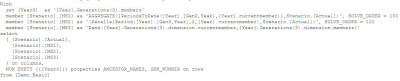# Archive for March 30th, 2009

## Oracle BI EE 10.1.3.4 and Hyperion Essbase 11.1.1.0 – Ago and ToDate functions – Custom MDX and Evaluate_Aggr – Achieving Time based Shifting and Period To Date Calculations

Posted by Venkatakrishnan J on March 30, 2009

One of the few topics that i have not covered so far in my BI EE and Essbase connectivity series is the support of Ago and ToDate functions. As you would probably know, Ago and ToDate are BI EE rpd specific functions which can basically help in achieving Time based shifting, Period To Date kind of calculations. Depending on the underlying database, BI EE automatically function ships these 2 functions into the corresponding relational database’s sql queries. I have covered this before for a relational source here and here. Now with the support for Essbase data sources in BI EE, Ago and ToDate functions are function shipped automatically to MDX specific functions. Lets try to understand what these 2 functions do and how they work on an Essbase data source.

1. Ago – This function basically calculates the value of the measure where the Time member would actually be shifted by an index specified in the Function.
2. ToDate – This function basically calculates the Period To Date value for every intersection of the Time dimension. i.e if you have Jan, Feb in your report, the Period to Date would calculate Jan, Jan + Feb.

For the above functions to work, you need a dimension marked as Time in your Essbase outline. So, lets start with the Demo->Basic cube.

Now, go to the BMM of this imported Essbase cube in the BI EE repository. Mark Year as the Time Dimension and also set the chronological key.

Once this is done, go to the fact table and create a custom column. This custom column would basically be used for doing periodic shift of prior 2 months. In the column enter the formula as shown below

```Ago("Demo"."Basic"."Actual" ,  "Demo"."Year"."Gen3,Year" , 2)
```

Now, include this column in the presentation layer and create a report as shown below. Ensure that the grain of the report matches the Ago function.

As you see, the Ago function automatically creates a custom metric calculating the value of the metric by shifting the month by 2 for every time member. Lets look at the MDX query.

```With
set [Year3] as '[Year].Generations(3).members'
member [Scenario].[MS1] as '(ParallelPeriod([Year].[Gen3,Year],2,[Year].currentmember),Scenario.[Actual])', SOLVE_ORDER = 100
select
{ [Scenario].[Actual], [Scenario].[MS1] } on columns,
NON EMPTY {{[Year3]}} properties ANCESTOR_NAMES, GEN_NUMBER on rows
from
[Demo.Basic]
```

As you see above, the Ago function is automatically function shipped to an MDX function called ParallelPeriod. The major drawback with Ago is that it hardcodes the ParallelPeriod function and hence all the capabilities of the ParallelPeriod function is not leveraged (like Hierarchy filters etc). If those are required, then Evaluate would have to be used. We shall see how this can be done later. Now that we are clear on how Ago works, lets move on to ToDate function. Go to the fact logical table in the BMM and add a custom column. In the custom column enter the formula shown below

``` TODATE(Demo.Basic.Actual, Demo."Year"."Gen2,Year")
```

If we look at the MDX query generated, you would notice that ToDate is automatically function shipped to PeriodsToDate MDX function.

```With
set [Year3] as '[Year].Generations(3).members'
member [Scenario].[MS1] as 'AGGREGATE({PeriodsToDate([Year].[Gen2,Year],[Year].currentmember)},Scenario.[Actual])', SOLVE_ORDER = 100
member [Scenario].[MS2] as '(ParallelPeriod([Year].[Gen3,Year],2,[Year].currentmember),Scenario.[Actual])', SOLVE_ORDER = 100
member [Scenario].[MS3] as 'Rank([Year].Generations(3).dimension.currentmember,[Year].Generations(3).dimension.members)'
select
{ [Scenario].[Actual], [Scenario].[MS1], [Scenario].[MS2], [Scenario].[MS3] } on columns,
NON EMPTY {{[Year3]}} properties ANCESTOR_NAMES, GEN_NUMBER on rows
from
[Demo.Basic]
```If you want to achieve the same functionality of Ago and Todate without actually using them, use them as Evaluate functions, as shown below

```EVALUATE_AGGR('AGGREGATE({PeriodsToDate([Year].[Gen2,Year],%1.dimension.currentmember)},Scenario.[Actual])' , "vejanaki-lap.in.oracle.com"."Demo".""."Basic"."Gen3,Year")
EVALUATE_AGGR('(ParallelPeriod([Year].[Gen3,Year],2,%1.dimension.currentmember),Scenario.[Actual])',"vejanaki-lap.in.oracle.com"."Demo".""."Basic"."Gen3,Year")
```

Its good that BI Server automatically function ships these functions back to Essbase in the form of MDX. But the drawback as noted above is the fact that one does not have the flexibility to support all the parameters of MDX. This hopefully should be available in the coming releases.

Posted in All Posts, EPM, Hyperion Essbase, OBI EE Plus | 7 Comments »